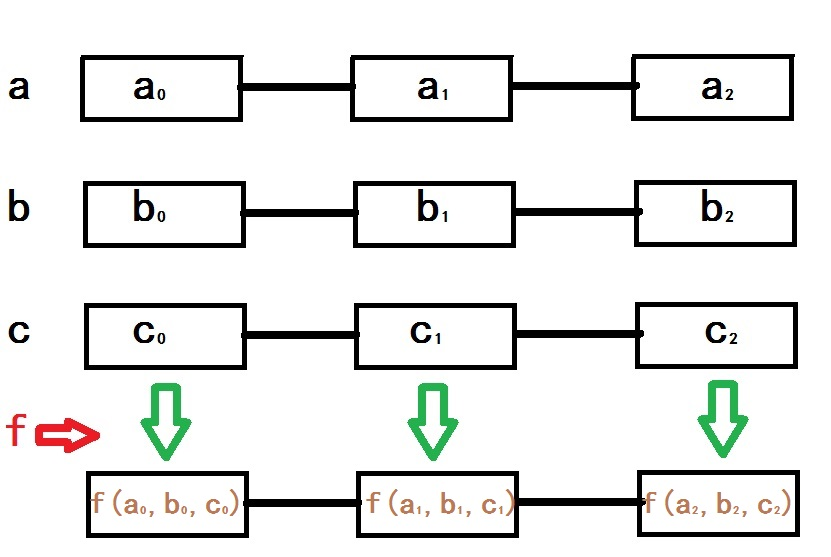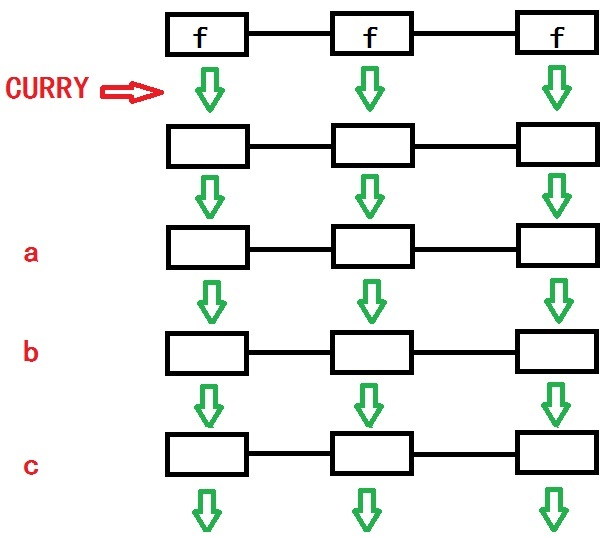版权申明：本文为博主窗户(Colin Cai)原创，欢迎转帖。如要转贴，必须注明原文网址

http://www.cnblogs.com/Colin-Cai/p/11329874.html

作者：窗户

QQ/微信：6679072

E-mail：6679072@qq.com

对于函数式编程来说，map/reduce/filter这几个算子非常重要，其中有的语言不是reduce而是fold，但功能基本一样，不过reduce的迭代一般只有一个方向，fold可能会分两个方向，这是题外话。

这篇文章就是来理解map的语义和实现，使用Scheme、Python、JS三种语言来解释一下这个概念。

map的语义

所谓算子，或者说高阶函数，是指输入或输出中带有函数的一种函数。一般情况下算子可能指输入中带有函数的情况，而对于输出中带有函数并带有输入参数信息的，我们很多情况下习惯叫闭包

map算子(高阶函数)是想同时处理n个长度相同的array或list等，它的输入参数中存在一个参数是函数。如图以一个简单的例子来演示map的作用，4个参数，一个参数是一个带有三个参数的函数f，另外三个参数是长度一样的list a、b、c。所有list依次按位置给出一个值，作为f的参数，依次得到的值组成的list就是map的返回值。

给个实际的例子：

map带上的参数中，函数是f:x,y->x-y，也就是的得到两个参数的差，带上两个list，分别是[10,9,8][1,2,3],则依次将(10,1)(9,2)(8,3)传给f，得到9,7,5，从而map返回的值是[9,7,5]

很多时候，map函数的处理是针对一个array/list的转换，从而看重面向对象编程的JS，其Array对象就有一个map方法。

map的一种实现

理解了map函数的语义之后，我们自然从过程式的思路明白了如何一个个的构造结果list的每个元素。但既然是函数式编程，一般来说，我们需要的不是过程式的思路，而是函数式的思路，最基本的思路是要去构造递归。

所谓递归，说白了就是寻找函数整体与部分的相似性。

我们还是用刚才的例子，用函数f:x,y->x-y，两个list为[10,9,8][1,2,3]，我们构造结果第一个数,需要先从[10,9,8]取出第一个元素10,从[1,2,3]中取出第一个元素1，用f:x,y->x-y作用得到9，此处[10,9,8][1,2,3]还剩下[9,8][2,3]尚未处理。而[9,8][2,3]的处理依然是map做的事情。于是这里就构造了一个递归：

1.处理每个list的第一个元素，得到结果list的第一个元素

2.map递归所有list的剩余部分，得到结果list的其他元素

3.拼接在一起，得到结果list

过程中，需要两个动作，一个对所有list取第一个元素，另一个是对所有list取剩余元素。单看这两个动作，共同点都是对所有list做的，不同点在于对每个list做的不同，一个是提取第一个元素，一个是提取剩余元素，于是我们这里就可以提取共性，也就是抽象。

我们先来做这个抽象，我们希望这样用，(scan s f)，带两个参数,一个是s是一个list，另一个是f，结果是一个和s等长度的list，它的元素和s的元素一一对应，由函数f转换而来。

和之前的map类似，这个也一样可以分为三部分：

1.处理s的第一个元素，为(f (car s))

2.scan递归s的剩余部分，为(scan (cdr s) f)

3.把两者用cons拼接在一起，为(cons (f (car s)) (scan (cdr s) f))

其实，这里少了一个边界条件，就是还得考虑s为空列的时候，返回也是空列。

于是scan的实现应该是

(define (scan  s f) (if (null? s) '() (cons (f (car s)) (scan (cdr s) f))))

同理，map也一样有边界条件，我们要考虑map所跟的那一组list都为空列的情况，这种情况返回也是空列。

于是map的实现应该是

(define (map f . s) (if (null? (car s)) '()

(cons

;处理每个list最开头的元素

(apply op (scan s car))

;递归处理剩余部分

(apply map2 op (scan s cdr)))))

apply是函数式编程支持语言里常用的功能，在于展开其最后一个为list的参数，比如apply(f, (1,2,3))也就是f(1,2,3)

然后，我们考虑Python的实现，因为序偶(pair)并非是Python的底层，我们需要用list拼接来实现，JS也一样。Python下用list的加号来实现拼接，为了简单起见，我们并不用生成器实现。

我们来模仿之前的Scheme,先实现scan函数。

scanlambda s,f : [] if len(s)==0 else [f(s)] + ([] if len(s)==1 else scan(s[1:],f))

Python的apply在早期版本里曾经存在过，后来都用*来取代了apply。比如f(*(1,2,3))在Python里就等同于f(1,2,3)

抛开这个不同，取代了之后，我们实现map如下

map = lambda f,*s : [] if len(s)==0 else [f(*scan(s, lambda x:x))] + map(f, *scan(lst, lambda x:x[1:]))

JS似乎比Python更看重面向对象，它的Array拼接用的是Array的concat方法，同时，它并没有Python那样的语法糖，不能像Python那样切片而只能用Array的slice方法，甚至于apply也是函数的方法的样子。另外，JS对可变参数的支持是使用arguments，需要转换成Array才可以切片。这些让我觉得似乎还是Python用起来更加顺手，不过这些特性让人看起来更加像函数式编程。另外，JS有很多框架，很多时候编程甚至看起来脱离了原始的JS。

所以以下map的实现虽然本质上和之前是一回事情，但写法看上去差别比较大了。

function map()
{
var op = arguments;
var scan = (s,f) => s.length==0?[]:[f(s)].concat(scan(s.slice(1),f));
var s = [].slice.call(arguments).slice(1);//先取得所有的list
return s.length==0 ? [] : [op.apply(this,scan(s, x=>x))].concat(map.apply(this,[op].concat(list_do(s, x=>x.slice(1)))));
}

函数式编程里，有一个概念叫柯里化，它将一个多参数的函数变成嵌套着的每层只有一个参数的函数。

return a+b+c

然后再定义另一个看起来有些诡异的函数

def  g(a):

def g2(b):

def g3(c):

return f(a,b,c)

return g3

return g2

这个函数g怎么用呢？

在此，我们希望设计一个函数来实现柯里化，curry(n ,f)，其中f为希望柯里化的函数，而nf的参数个数。

h = lambda a,b : lambda c : add(a, b, c)

那么 g = curry(2, h)

为了对于所有的curry都可以如此递归，要考虑之前讨论的不定参数，Python下也就是用*实现，而Scheme用apply，重写h函数如下：

h = lambda  *s : lambda c : add(*(s+(c,)))

于是，得到curry的Python实现：

def  curry(n, f):

return f if n==1 else curry(n-1lambda *s : lambda c : f(*(s+(c,))))

再者，curry函数本身一样可以柯里化，

于是，还可以写成

不断对curry柯里化，以下结果都是一样的，

...

Scheme的版本也就很容易根据上述Python的实现来改写，

(define  (curry n f)  (if (= n 1) f (curry (- n 1) (lambda s (lambda  (a) (apply f (append s (list a))))))))

JS的版本中，也需要用到函数的方法apply来实现不定参数，以及数组的concat方法来实现数组拼接。

function curry(n, f)
{
return n==1 ? f : curry(n-1, function () {return a => f.apply(this, [].slice.apply(arguments).concat([a]))});
}

这里引入柯里化的原因，自然也是为了实现map

我们这样去想，我们先把map的参数f柯里化，然后依次一步步的每次传一个参数，巧妙的利用闭包传递信息，直到最终算出结果。之前实现的scan对于每个元都采用相同的函数处理，这里要有所区别，每个数据都有自己独立的函数来处理，所以处理的函数也组成一个相同长的list。

与之前几乎相同，只是f成了一个list。

(define  (scan s f) (if  (null?  s) '() (cons ((car  f) (car  s)) (scan (cdr  s) (cdr  f)))))

而对于(map op . s)的定义，我们首先要把op柯里化了，(curry (length  s) op)，因为op会有(length s)个参数。

同时，最终的结果是(length (car s))个元素的list，所以是(length (car  s))个值按s来迭代，所以迭代初始值是(make-list (length (car  s)) (curry (length  s) op))

最后，我们顺着s从左到右的方向按照scan迭代一圈即可，我们用R6RS的fold-left来做这事。

(define (map  op . s) (fold-left  scan (make-list (length (car s)) (curry (length  s) op)) s))

Python下，scan也很容易修改：

scanlambda s,f : [] if  len(s)== else [f(s)] + ([] if  len(s)== else scan(s[1:],f[1:]))

Python下的reduce和Scheme的fold-left语义基本一致，再者Scheme下的make-list在Python下用个乘号就简单实现了。

map = lambda f,*s : reduce(scan, s, [curry(len(s), f)] * len(s))

Python3下reduce在functools里，需要事先import

from functools import reduce

JS下的scan倒是修改起来没有什么难度，JS下的reduce是Array的一个方法，make-list是用一个分配好长度的Array用fill方法实现，JS的确太面向对象了。

function map()
{
var scan = (f,s) => s.length==0 ? [] : [(f)(s)].concat(scan(f.slice(1),s.slice(1)));
return [].slice.call(arguments).slice(1).reduce(scan ,(new Array(arguments.length)).fill(mycurry(arguments.length-1, arguments)));
}

我们可以考虑map的柯里化，如果我们可以先得到map的柯里化，那么就很容易得到最终的结果。

说白了，也就是我希望这样：

(define (map op . s)

(foldl (lambda (n r) (r n)) map-currying-op s)

)

(curry (+ 1 (length s)) map) 是对map的柯里化，map-currying-op也就是要实现((curry (+ 1 (length s)) map) op)

最开始的时候，是意识到构造这个柯里化与之前scan有一定的相似性，需要利用其数据的list形成闭包，从而抽象出curry-map这个高阶函数。再者闭包所封装的数据中不仅仅有各层运算中的list，还需要带有计算层次的信息，因为最终的一次scan的结果得到的并不是函数，而是map的结果了，将计算层次和list形成pair，计算层次每往后算一个list，则减1，直到变成1了，下一步得到的就不再是闭包。

(define (map op . s)

(define scan

(lambda (s f)

(if (null? s)

'()

(cons ((car f) (car s)) (scan (cdr s) (cdr f))))))

(define curry-map

(lambda (x)

(if (= (car x) 1)

(lambda (s) (scan s (cdr x)))

(lambda (s)

(curry-map

(cons

(- (car x) 1)

(scan s (cdr x))))))))

(define map-currying-op

(curry-map

(cons

(length s)

(make-list (length (car s)) (curry (length s) op)))))

(fold-left (lambda (n r) (r n)) map-currying-op s)

)

上述实现就是通过map的柯里化来实现map,可能比较复杂而拗口，我在构造实现的时候也一度卡了壳，这个很正常，形式化的世界里的确有晦涩的时候。

另外，实际上这里curry-map并不是对map的柯里化，只是这样写更加整齐一些，其实也可以改变一下，真正得到map的柯里化，这个只是一个小小的改动。

(define curry-map
(lambda (x)
(if (pair? x)
(if (= (car x) 1)
(lambda (s) (scan s (cdr x)))
(lambda (s)
(curry-map
(cons
(- (car x) 1)
(scan s (cdr x))))))
(curry-map
(cons
(length s)
(make-list (length (car s)) (curry (length s) x)))))))
(define map-currying-op
(curry-map op))

有兴趣的朋友可以分析一下这一节的所有代码，在此我并不给出Python和JS的实现，有兴趣的可在明白了之后可以自己来实现。

以上的实现可以帮助我们大家去从所使用语言的内部去理解这些高阶函数。但实际上，这些作为该语言基本接口的map/reduce/filter等，一般是用实现这些语言的更低级语言来实现，如此实现有助于提升语言的效率。比如对于Lisp，我们在学习Lisp的过程能中，可能会自己去实现各种最基本的函数，甚至包括cons/car/cdr，但是要认识到现实，在我们自己去实现Lisp的解释器或者编译器的时候，还是会为了加速，把这些接口放在语言级别实现里。

posted on 2019-08-14 17:34  窗户  阅读(1010)  评论(0编辑  收藏  举报